# 全球名校课程作业分享系列(4)--斯坦福计算机视觉与深度学习CS231n之双层神经网络完成图像多分类

### 2 知识点

#### 2.1 神经元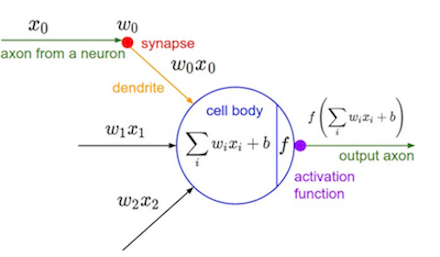iwixi+b

f(iwixi+b)

σ(x)=1/(1+ex)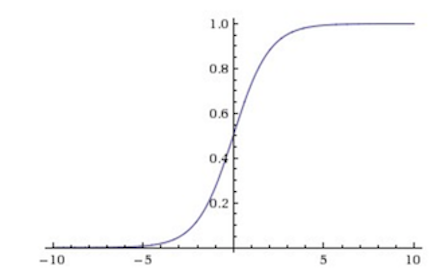y=sigmoid(w⃗ Tx⃗ )

f(x)=max(0,x)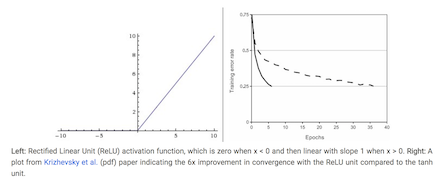ReLu在实践中被证明的优点是它在随机梯度下降法中收敛效果非常好，此外它的梯度值非常简单。但它的缺点也非常明显，就是当它的梯度为0的时候，这个神经元就相当于把从后面传过来的梯度值都变成了0，使得某些变量在梯度下降法法中不再变化。cs231n官网笔记里有关于不同激活函数的详细介绍，大家有兴趣可以看一下：http://cs231n.github.io/neural-networks-1/

### 2.2 神经网络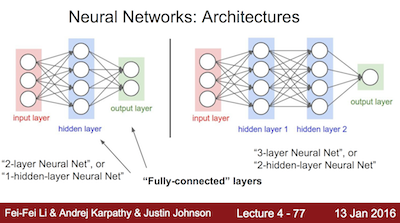W=w⃗ T1w⃗ T2w⃗ T3w⃗ T4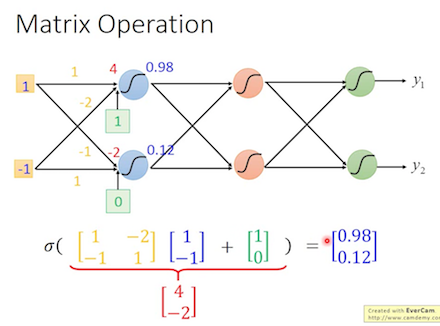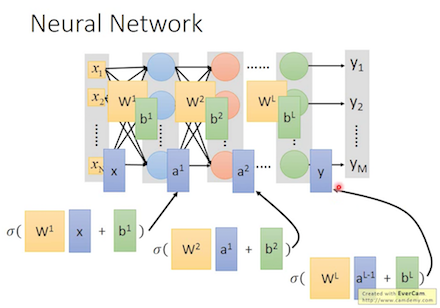#### 2.3 反向传播算法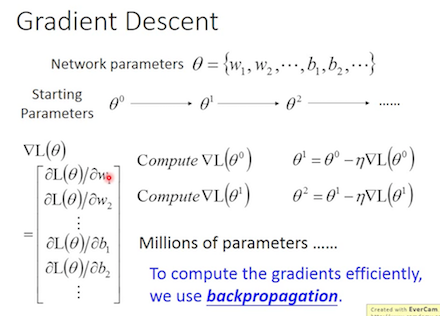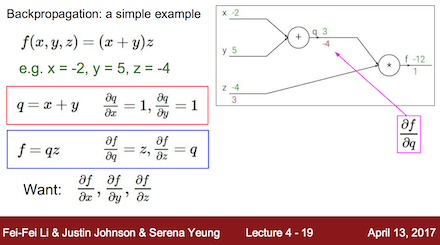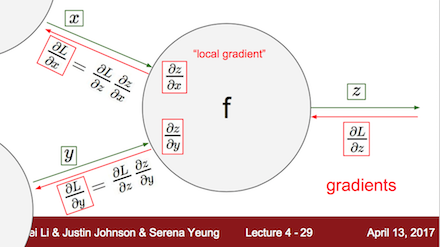fx=fqqx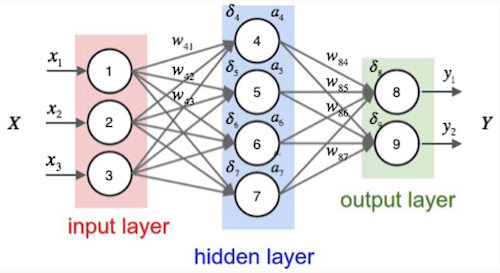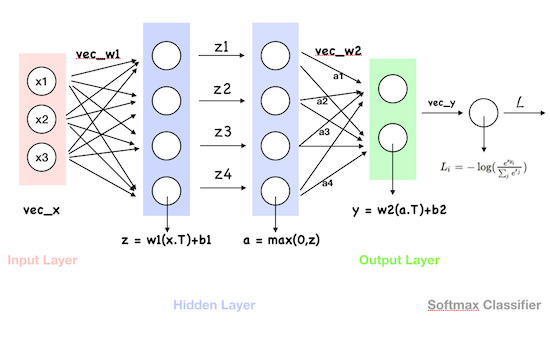Lifk=pk?(yi=k)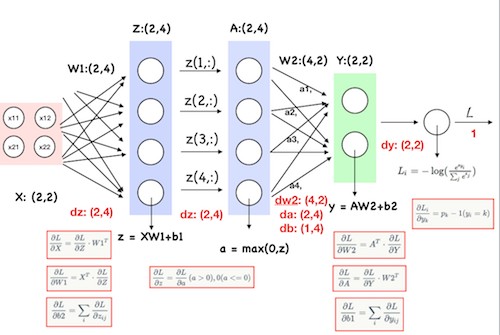### 3 代码解析

#### 3.1 初始化代码


# 导入模块和库
import numpy as np
import matplotlib.pyplot as plt

from cs231n.classifiers.neural_net import TwoLayerNet

from __future__ import print_function

%matplotlib inline
plt.rcParams['figure.figsize'] = (10.0, 8.0) # set default size of plots 设置图表的默认大小
plt.rcParams['image.interpolation'] = 'nearest'
plt.rcParams['image.cmap'] = 'gray'

# 自动加载外部的模块

def rel_error(x, y):
""" returns relative error """
return np.max(np.abs(x - y) / (np.maximum(1e-8, np.abs(x) + np.abs(y))))

#### 3.2 加载测试数据


#  创建一个小的网络和测试数据来检查你的实现。注意我们用了随机种子来帮助实现实验的可重复性

input_size = 4
hidden_size = 10
num_classes = 3
num_inputs = 5

def init_toy_model():
np.random.seed(0)
return TwoLayerNet(input_size, hidden_size, num_classes, std=1e-1)

def init_toy_data():
np.random.seed(1)
X = 10 * np.random.randn(num_inputs, input_size)
y = np.array([0, 1, 2, 2, 1])
return X, y

net = init_toy_model()
X, y = init_toy_data()

#### 3.3 前向传播：计算分值

#插播neural.net.py文件中的第一部分代码
z1 = X.dot(W1) + b1
a1 = np.maximum(0, z1)
scores = a1.dot(W2) + b2
#插播结束
scores = net.loss(X)
print(scores)
print()
print('correct scores:')
correct_scores = np.asarray([
[-0.81233741, -1.27654624, -0.70335995],
[-0.17129677, -1.18803311, -0.47310444],
[-0.51590475, -1.01354314, -0.8504215 ],
[-0.15419291, -0.48629638, -0.52901952],
[-0.00618733, -0.12435261, -0.15226949]])
print(correct_scores)
print()

# 差值应该非常小，小于1e-7
print('Difference between your scores and correct scores:')
print(np.sum(np.abs(scores - correct_scores)))


[[-0.81233741 -1.27654624 -0.70335995]
[-0.17129677 -1.18803311 -0.47310444]
[-0.51590475 -1.01354314 -0.8504215 ]
[-0.15419291 -0.48629638 -0.52901952]
[-0.00618733 -0.12435261 -0.15226949]]
correct scores:
[[-0.81233741 -1.27654624 -0.70335995]
[-0.17129677 -1.18803311 -0.47310444]
[-0.51590475 -1.01354314 -0.8504215 ]
[-0.15419291 -0.48629638 -0.52901952]
[-0.00618733 -0.12435261 -0.15226949]]
Difference between your scores and correct scores:3.68027207459e-08

#### 3. 前向传播：计算损失值

#插播neural.net.py文件中的第二部分代码
exp_scores = np.exp(scores)
probs = exp_scores / np.sum(exp_scores, axis=1, keepdims=True)
#插播结束
loss, _ = net.loss(X, y, reg=0.05)
correct_loss = 1.30378789133

# 应该非常小，小于1e-12
print('Difference between your loss and correct loss:')
print(np.sum(np.abs(loss - correct_loss)))

Difference between your loss and correct loss:
0.0189654196061

#### 3.4 反向传播

# 插播neural.net.py文件中的第三部分代码
# 计算分值的梯度
dscores = probs
dscores[range(N),y] -= 1
dscores /= N

# W2 和 b2

# 反向传播里第二个隐藏层
dhidden = np.dot(dscores, W2.T)

# 激活函数ReLu的梯度
dhidden[a1 <= 0] = 0

# 关于W1和b1的梯度

# 加上正则化梯度的部分
#插播结束
from cs231n.gradient_check import eval_numerical_gradient

# 使用数值梯度法检查反向传播的实现。如果你的实现正确的话，每一个W1，W2，b1和b2的分析梯度和数值梯度之间的差值应该小于1e-8
loss, grads = net.loss(X, y, reg=0.05)

# 这些都应该小于1e-8
f = lambda W: net.loss(X, y, reg=0.05)


W1 max relative error: 4.090896e-09
W2 max relative error: 3.440708e-09
b2 max relative error: 4.447646e-11
b1 max relative error: 2.738421e-09

#### 3.5 训练神经网络

#下面插播neural.net.py文件里train函数的缺失代码
sample_indices = np.random.choice(np.arange(num_train), batch_size)
X_batch = X[sample_indices]
y_batch = y[sample_indices]
##########################################

#下面是neural.net.py文件里predic函数的代码
z1 = X.dot(self.params['W1']) + self.params['b1']
a1 = np.maximum(0, z1) # pass through ReLU activation function
scores = a1.dot(self.params['W2']) + self.params['b2']
y_pred = np.argmax(scores, axis=1)
#插播结束
net = init_toy_model()
stats = net.train(X, y, X, y,
learning_rate=1e-1, reg=5e-6,
num_iters=100, verbose=False)

print('Final training loss: ', stats['loss_history'][-1])

# 画出迭代过程的损失值变化图像
plt.plot(stats['loss_history'])
plt.xlabel('iteration')
plt.ylabel('training loss')
plt.title('Training Loss history')
plt.show()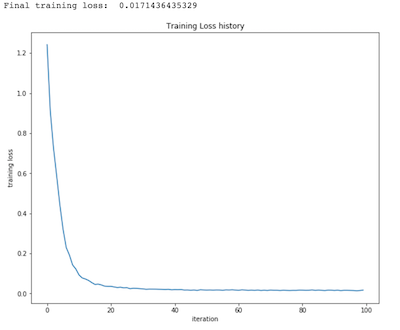#### 3.6 加载CIFAR-10图像数据

from cs231n.data_utils import load_CIFAR10

def get_CIFAR10_data(num_training=49000, num_validation=1000, num_test=1000):
"""
Load the CIFAR-10 dataset from disk and perform preprocessing to prepare
it for the two-layer neural net classifier. These are the same steps as
we used for the SVM, but condensed to a single function.
"""

# 加载CIFAR-10数据
cifar10_dir = 'cs231n/datasets/cifar-10-batches-py'
X_train, y_train, X_test, y_test = load_CIFAR10(cifar10_dir)

# 从数据集中取数据子集用于后面的练习
mask = list(range(num_training, num_training + num_validation))

# 标准化数据：先求平均图像，再将每个图像都减去其平均图像
mean_image = np.mean(X_train, axis=0)
X_train -= mean_image
X_val -= mean_image
X_test -= mean_image

# 将所有的图像数据都变成行的形式
X_train = X_train.reshape(num_training, -1)
X_val = X_val.reshape(num_validation, -1)
X_test = X_test.reshape(num_test, -1)

return X_train, y_train, X_val, y_val, X_test, y_test

# 调用该函数以获取我们需要的数据，查看数据集的大小
X_train, y_train, X_val, y_val, X_test, y_test = get_CIFAR10_data()
print('Train data shape: ', X_train.shape)
print('Train labels shape: ', y_train.shape)
print('Validation data shape: ', X_val.shape)
print('Validation labels shape: ', y_val.shape)
print('Test data shape: ', X_test.shape)
print('Test labels shape: ', y_test.shape)


Train data shape: (49000, 3072)
Train labels shape: (49000,)
Validation data shape: (1000, 3072)
Validation labels shape: (1000,)
Test data shape: (1000, 3072)
Test labels shape: (1000,)

#### 3.7 训练神经网络

input_size = 32 * 32 * 3
hidden_size = 50
num_classes = 10
net = TwoLayerNet(input_size, hidden_size, num_classes)

# 训练网络
stats = net.train(X_train, y_train, X_val, y_val,
num_iters=1000, batch_size=200,
learning_rate=1e-4, learning_rate_decay=0.95,
reg=0.25, verbose=True)

# 在验证集上进行预测
val_acc = (net.predict(X_val) == y_val).mean()
print('Validation accuracy: ', val_acc)

iteration 0 / 1000: loss 2.302762
iteration 100 / 1000: loss 2.302358
iteration 200 / 1000: loss 2.297404
iteration 300 / 1000: loss 2.258897
iteration 400 / 1000: loss 2.202975
iteration 500 / 1000: loss 2.116816
iteration 600 / 1000: loss 2.049789
iteration 700 / 1000: loss 1.985711
iteration 800 / 1000: loss 2.003726
iteration 900 / 1000: loss 1.948076
Validation accuracy: 0.287

#### 3.8 调试训练结果


# 绘制损失值
plt.subplot(2, 1, 1)
plt.plot(stats['loss_history'])
plt.title('Loss history')
plt.xlabel('Iteration')
plt.ylabel('Loss')

plt.subplot(2, 1, 2)
plt.plot(stats['train_acc_history'], label='train')
plt.plot(stats['val_acc_history'], label='val')
plt.title('Classification accuracy history')
plt.xlabel('Epoch')
plt.ylabel('Clasification accuracy')
plt.show()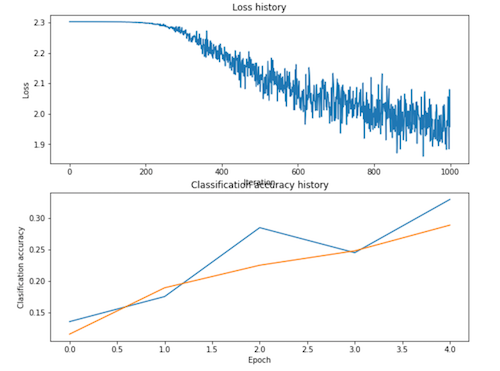from cs231n.vis_utils import visualize_grid

# 可视化网络的权重
def show_net_weights(net):
W1 = net.params['W1']
W1 = W1.reshape(32, 32, 3, -1).transpose(3, 0, 1, 2)
plt.gca().axis('off')
plt.show()
show_net_weights(net)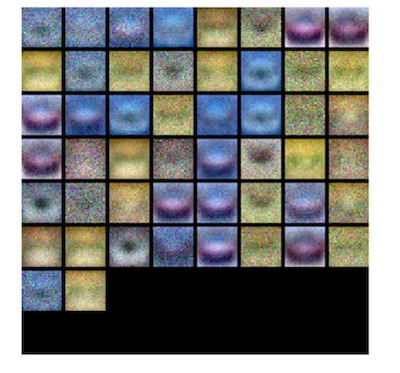#### 3.9 调试你的超参数

best_net = None # store the best model into this
#将最好的模型放进这里

# 使用验证集调整超参数。在best_net变量中存储最好模型的模型参数。为了帮助调试你的网络，使用类似于上面用过的可视化方法可能会有帮助; 这里的可视化结果与上面调试较差的网络中得到的可视化结果会有显著的质的差异。

best_val = -1
best_stats = None
learning_rates = [1e-2, 1e-3]
regularization_strengths = [0.4, 0.5, 0.6]
results = {}
iters = 2000 #100
for lr in learning_rates:
for rs in regularization_strengths:
net = TwoLayerNet(input_size, hidden_size, num_classes)

# Train the network
stats = net.train(X_train, y_train, X_val, y_val,
num_iters=iters, batch_size=200,
learning_rate=lr, learning_rate_decay=0.95,
reg=rs)

y_train_pred = net.predict(X_train)
acc_train = np.mean(y_train == y_train_pred)
y_val_pred = net.predict(X_val)
acc_val = np.mean(y_val == y_val_pred)

results[(lr, rs)] = (acc_train, acc_val)

if best_val < acc_val:
best_stats = stats
best_val = acc_val
best_net = net

# Print out results.
for lr, reg in sorted(results):
train_accuracy, val_accuracy = results[(lr, reg)]
print ("lr ",lr, "reg ", reg, "train accuracy: ", train_accuracy, "val accuracy: ", val_accuracy)

print ("best validation accuracy achieved during cross-validation: ", best_val)
#################################################################################
#                               END OF YOUR CODE                                #
#################################################################################

lr 0.001 reg 0.4 train accuracy: 0.516408163265 val accuracy: 0.48
lr 0.001 reg 0.5 train accuracy: 0.53006122449 val accuracy: 0.479
lr 0.001 reg 0.6 train accuracy: 0.520591836735 val accuracy: 0.497
lr 0.01 reg 0.4 train accuracy: 0.100265306122 val accuracy: 0.087
lr 0.01 reg 0.5 train accuracy: 0.100265306122 val accuracy: 0.087
lr 0.01 reg 0.6 train accuracy: 0.100265306122 val accuracy: 0.087
best validation accuracy achieved during cross-validation: 0.497


# 可视化最好的神经网络的权重
show_net_weights(best_net)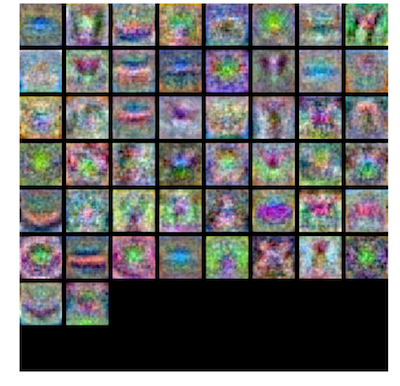#### 3.10 在测试集上测试

test_acc = (best_net.predict(X_test) == y_test).mean()
print('Test accuracy: ', test_acc)

Test accuracy: 0.474

©️2019 CSDN 皮肤主题: 编程工作室 设计师: CSDN官方博客